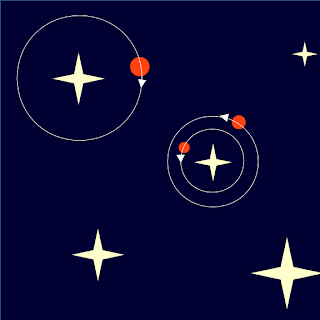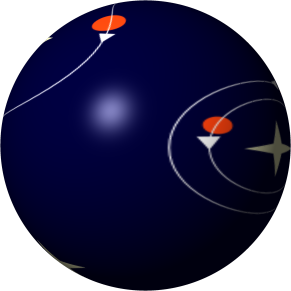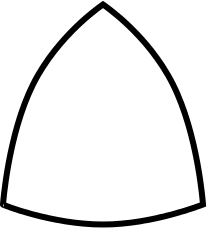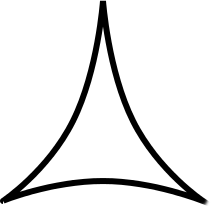## Thursday, July 24, 2014

### Shape of the Universe

At the moment I'm reading A Universe From Nothing by Lawrence Krauss and there's a section which talks about the shape of the universe. So what does it mean for the universe to have a shape?

Let's imagine a flat 2D universe. What this means is that things can move left, right, forward, and backward but not up and down. Everything is flat, as shown in this picture:Now think of this universe as a bed sheet. The sheet may be laid on the floor, in which case it would have a flat shape, but it might be placed on some curved surface, such as a ball.Is there a way for the inhabitants of such a spherical universe to know that their universe is a sphere? Yes. By using triangles. A triangle in a flat universe follows Euclidian geometry rules, such as that the sum of its angles add up to 180 degrees, but what about on the surface of a sphere?

On a sphere, it is actually possible to draw a triangle consisting of three 90 degree angles. Divide the sphere into 8 quadrants (break it into two halves, then each halve into another two halves, and each of those into yet another two halves). Each quadrant is a triangle consisting of only 90 degree angles. How can this happen? Its because lines on a sphere that diverge tend to reconverge at some point, which doesn't happen on a flat plane.

So, if the inhabitants of this spherical universe were to try to construct a triangle (which has to be really big) and find that each angle is 90 degrees, then they would have proven that they live in a spherical universe.

There are other shapes of the universe that are possible, such as the saddle shape below, also from Wikipedia:

The inhabitants of a saddle shaped universe can tell that their universe is a saddle shape and not a sphere because lines tend to diverge rather than converge, and so the triangle will have angles which are too small, rather than too big.

This is what the inhabitants would see if they drew a triangle on a...

spherical universe:flat universe:You might be asking about other weird shapes that the universe can be in. These shapes were derived from general relativity and depend on the mass of the universe. Different masses give rise to variations of these three shapes (how curved the triangles are) so this is all we will consider.

Now there is no actual need to measure all the angles of the triangle in order to see if they add up to 180 degrees. A more practical solution might be to stand at one of the points, say the top point, put a ruler at the bottom of the triangle a known distance away from you, and check how wide you have to open your field of vision in order to see the whole ruler. From the above three triangles you will have noticed that the top corner of each triangle has a different angle in order to achieve the same base length. In a spherical universe you'd have to have a wider top angle in order to achieve a 1 km base length, whilst in a saddle shaped universe you'd have to have a narrower top angle in order to achieve a 1 km base length. By measuring how wide the angle of vision has to be in order to see a ruler of a known length, a known distance away, the inhabitants of the universe can know what shape their universe is.Of course there is no ruler readily available for us to use, especially not one that is big enough and far enough to measure subtle curvatures, and of course the universe is not two dimensional. Let's deal with these one at a time.

Does it matter than the universe is three dimensional? No, these same shapes can happen in any number of dimensions, as long as there is another dimension along which to curve. So can there be four dimensions? Determining if our universe is flat or not can answer that question. But if there is a fourth dimension, then we wouldn't be aware of it, just like the inhabitants of the flat universe would not be aware of a third dimension as they can only travel along the surface and cannot jump up or dig down. In the same way, we can only travel along our three dimensional space but we cannot move along a fourth dimension so we never needed to be aware of it. One thing is for sure however, 3D triangles still behave in the same way on a 4D sphere.

So how do we use the ruler experiment to determine the shape of the universe? Obviously we won't be using an actual ruler, but we can use something that is almost as good. The universe is expanding as we speak and everything is going further apart from each other, which means that in the past it was smaller and everything was closer together. What does this mean? That's not all. If you look far away, you will be seeing things from the past. This is because light is not instantaneous but travels at a constant speed, which means if you're looking at something which is so far away that the light reaching your eyes took 1000 years of travel, you are seeing what it looked like 1000 years ago. The oldest thing you can see is called the cosmic microwave background radiation. This is when the universe was 300,000 years old and was so dense that it was an opaque blob (light could not pass). This means that we cannot "see" past this 300,000 year mark, it is the oldest thing we can see in the universe.

Now here comes the crux of the matter: Gravity also travels at the speed of light. If the sun suddenly disappeared, the Earth would still be revolving around it as if nothing happened for 8 minutes. After 8 minutes, the Earth would fling out of orbit. This is because the Earth is 8 light minutes away from the sun. Since this Cosmic Microwave Background Radiation is only 300,000 years old, anything further apart than 300,000 light years would not be gravitationally affected. In other words, if there are two blobs that are more than 300,000 light years apart would not be gravitationally attracted to each other. This sets our ruler, a 300,000 light year long ruler. All you have to do is see how far apart from each other blobs have to be in order to not be attracted to each other. This is translated as checking how packed together the blobs were; with gravity attracting only blobs that were closer than 300,000 light years together, there will be a certain density of blobs that can be found by computer simulation.

So the trick is to check how wide an angle of vision must be used in order to see a certain number of blobs. If the universe is spherical, a narrower angle must be used, if saddle shaped, a wide angle must be used, and if flat, an angle in between must be used. Another approach was taken however in the BOOMERanG experiment. Rather than check what angle must be used to see a number of blobs, the number of blobs for a particular angle was found, together with the actual number of blobs seen, and illustrated in here (see the last picture).

The actual observation is very similar to what should have been seen if it was flat. This is evidence that the universe is, in fact, "flat", that is it is not curved upon some fourth dimensional curve.